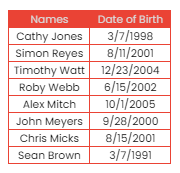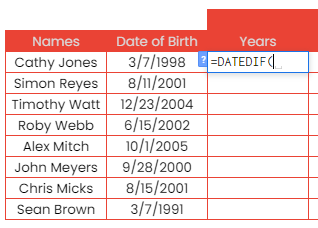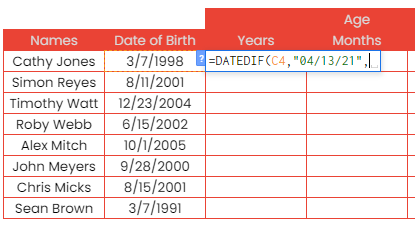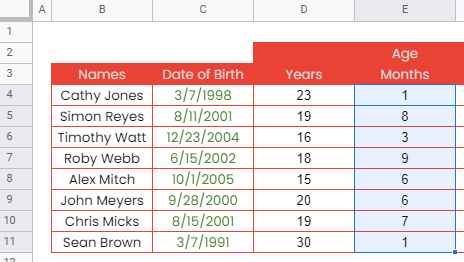# How to Use DATEDIF Function in Google Sheets

The DATEDIF function in Google Sheets is useful if you want to know how far two different given dates are from each other.

Meaning, the DATEDIF function calculates the difference between two date values. The results can be returned either as the number of days, months, or years.

The rules for using the DATEDIF function in Google Sheets are as follows:

• The function will return a #NUM! error if the date in the first argument is after the date in the second argument.
• Like most functions, the DATEDIF function will return a #N/A error if not all 3 arguments are provided.
• Constant values as arguments need to be enclosed with quotation marks (“).

Let’s take an example.

Maine, a school registrar, has been so busy with the upcoming school opening. She’s been trying to figure out the students’ age using the only available information, which is the date of birth of each student. See the tables below:Luckily, Maine has been a Sheetaki subscriber since 2020 and she was able to read our article entitled How To Calculate Days Between Dates In Google Sheets, where we talked about using the DATEDIF function. She was able to compute their students’ age using the DATEDIF function. Please see below:Easy-peasy, right?

The age values shown above are the difference between the given date of birth and the date today, which is 04/12/2021, in terms of years.

What if Maine also wants to include the months?

She made a few tweaks to the DATEDIF function and applied it to her given dates values. See the results below:Notice how the results became more accurate this time.

Maine didn’t stop playing around with the DATEDIF function. She made another tweak and was able to include the days in the age format. See how brilliant she and the DATEDIF function below:See how, using 1 function, we can calculate the exact difference between now and the given dates of birth.

Watch out for a more advanced tutorial and examples on how you can use the DATEDIF function in the coming weeks. Be sure to subscribe to be notified.

Awesome! Let’s begin getting to know more about our DATEDIF function in Google Sheets.

## The Anatomy of the DATEDIF Function

So the syntax (the way we write) the DATEDIF function is as follows:

```=DATEDIF(start_date,end_date,unit)
```

Let’s dissect this thing and understand what each of these terms means:

• = the equal sign is just how we start any function in Google Sheets. It is how Google Sheets understand that we are asking it to either do computation or use a function.
• DATEDIF() this is our DATEDIF function. It will take arguments and calculate the difference between the two provided date values.
• start_date is the date at which to start the calculation.
• end_date is the date at which to end the calculation.
• unit is the type of result that you want the function to return. The options are ‘Y’, ‘M’, ‘D’, ‘YM’, ‘YD’, or ‘MD’.

## A Real Example of Using DATEDIF Function

In our previous article about the DATEDIF function, we explained and provided samples of date difference in terms of years, months and days by using “Y”, “M”, and “D” as the unit. In this article, we will focus on other units such as “YD”, “YM” and “MD”.

Take a look at our students’ record example below to see how the DATEDIF function is used in Google Sheets.The values in the Years column were obtained using the following syntax:

=DATEDIF(C4,“04/12/2021”,“Y”)

The first argument used is the cell address of the date of birth of the first student, while the second argument is a constant of the current date.

Notice the third argument that we passed on the function is the capital letter ‘Y’ enclosed with quotation marks.

The syntax simply instructed the Google Sheets to perform a calculation that would return the difference between the first and second argument in the unit ‘Y’, which means in years. Hence, the result was 23. So basically, there are 23 years between 3/7/1998 and 4/12/2021. You can validate that by subtracting 1998 from 2021. The 23rd year mark took place on 3/7/2021.

The values in the Months column were obtained using the following syntax:

=DATEDIF(C4,“04/12/2021”,“YM”)

The same first and second arguments were passed on to our function here. The only difference is the third argument, which is the unit ‘YM’.

Like the first syntax, our function here will calculate the difference between the two date values. It will return the number of months elapsed after the number of years shown in the ‘Y’ unit, or 23 in our first syntax.

Ignoring the years, with the ‘YM’ as the unit in the DATEDIF function, it will return the number of completed months after subtracting the full years. In our example, it returned the value 1 month.

This means that, after the 23rd year mark on 3/7/2021 from 3/7/1998, 1 month has since elapsed. You can validate that since it’s almost over a month from 3/7/2021 to 4/12/2021.

The values in the Days column were obtained using the following syntax:

=DATEDIF(C4,“04/12/2021”,“MD”)

The difference has been calculated to return the results in the ‘MD’ unit. It is the number of days elapsed after the number of months shown with the “M” or “YM” unit.

In our example, the return value is 5 days, which means after subtracting the whole months between the two given date values, there were only 5 days elapsed.

You can validate that by checking that, after the 23rd year mark on 3/7/2021 from 3/7/1998, 1 month and 5 days have already elapsed by 4/12/2021. The 1-month mark is on 4/7/2021. Since it’s already 4/12, 5 days have gone by since then.

Lastly, the use for the ‘YD’ as the unit in our DATEDIF function is to return the number of days after subtracting the whole year. See a separate example below:The syntax used is as follows:

=DATEDIF(C5,“04/12/2021”,“YD”)

The return value was 36, which means there have been 36 days elapsed since the 23rd year mark from 3/7/1998 to 4/12/2021. This means that from 3/7/2021 up to 4/12/2021, there are 36 days in between.

Just like our two table examples above, the unit ‘YM’, ‘MD’, and ‘YD’ are best partnered with the units ‘Y’ and ‘M’ to make a more meaningful data presentation for users.

You may make a copy of the spreadsheet using the link I have attached below.

## How to Use DATEDIF Function in Google Sheets

1. Click on any cell to make it the active cell. For this guide, I will be selecting D4, where I want to show my result.1. Next, type the equal sign ‘=‘ to begin the function and then follow it with the name of the function, which is our ‘datedif‘ (or ‘DATEDIF‘, not case sensitive like our other functions).1. Type open parenthesis ‘(‘ or simply hit Tab key to let you use that function.1. Now the exciting part! Let’s give our function its first argument, the start_date. Click the cell C4, or manually type in C4.1. To let the Google sheet know that we’re done typing our first argument, we should now type in the delimiter or the character that separates each argument on a function. In this case, type comma,’.1. Type in our second argument, which is the end_date. Similar to the first argument, the 2nd argument can be referenced using a cell address. In this example, we will use the date today and pass it directly in the function. Type in “04/13/21” follow it with the comma “,”.1. Lastly, we will now pass to the function the third argument, which is the unit. In this scenario, we need the difference in years. So, type in “Y”.1. Finally, hit your Enter or Tab key. Cell D4 will now show you the result or the return value of the DATEDIF function.1. Copy the formula down to the remaining rows.1. D4 to D11 will now show you the difference between the date of birth values and the date today in terms of years.1. Let’s now move on to getting the remaining months after subtracting the years of the two given date values. We can do this by using the same function, first and second argument. As for the unit, we will be using “YM”, instead of “Y”.1. As for the third argument, type in “YM”.1. Finally, hit your Enter or Tab key. Cell E4 will now show you the result or the return value of the DATEDIF function.1. Copy the formula down to the remaining rows.1. E4 to E11 will now show you the difference between the date of birth values and the date today in terms of months after subtracting the full years.1. Let’s now get the remaining days after subtracting the months of the two given date values. We can do this by using the same function, first and second arguments. As for the unit, we will be using “MD”, instead of “YM”.

1. In cell F4, follow steps 2-6. You should have the same syntax below:1. Type in “MD” as the third argument.1. Hit your Enter or Tab key. Cell F4 will now show you the result or the return value of the DATEDIF function.1. Copy the formula down to the remaining rows.1. F4 to F11 will now show you the difference between the date of birth values and the date today in terms of days after subtracting the full months.1. Take a look at our final results below:That’s pretty much it. You can now use the DATEDIF function in Google Sheets together with the other numerous Google Sheets formulas to create even more powerful formulas that can make your life much easier.Our goal this year is to create lots of rich, bite-sized tutorials for Google Sheets users like you. If you liked this one, you'll love what we are working on! Readers receive ✨ early access ✨ to new content.

##### You May Also Like## How to Use IF Functions in Google Sheets

The IF function is useful if you want to test whether a certain condition is true or false.…## How to Count the Number of Words in Google Sheets

Knowing how to count the number of words in Google Sheets is useful if you need to know…## How to Use the YEAR Function in Google Sheets

The YEAR function in Google Sheets is useful to return the year from any given unit of time,…## How to Count Rows Between Two Values in Google Sheets

Knowing how to count rows between two values in Google Sheets is useful if you want to find…